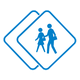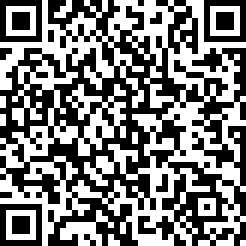#### ACT American College Testing (Exam 6)

created by Fisher BRink (@fisher) at Feb. 8, 2016
• Balloons are sold according to the chart above. If a customer buys one balloon at ...

• In the graph above, ABCD is a square. What are the coordinates of point B?

• Line - 5 is perpendicular to line

• If 30% of r is equal to 75% of s, what is 50% of s if r = 30?

• A dormitory now houses 30 men and allows 42 square feet of space per man. If five ...

• Rob has six songs on his portable music player. How many different four-song order...

• The statement "Raphael runs every Sunday" is always true. Which of the following s...

• In the diagram above, lines EF and GH are parallel, and line AB is perpendicular t...

• Which of the following is the midpoint of a line with endpoints at (2,8) and (8,0)?

• If

• A music store offers customized guitars. A buyer has four choices for the neck of ...

• Which of the following is the set of positive factors of 12 that are NOT multiples...

• The graph of is shown above. How many values can be found for ?

• Which of the following is the vertex of the parabola which is the graph of the equ...

• If the statement "No penguins live at the North Pole" is true, which of the follow...

• The pie chart above shows the distribution of video rentals from Al's Video Vault ...

• If the circumference of the circle in the diagram above is 20 units, what is the a...

• What is the slope of the line 3y = 12x 3?

• An empty crate weighs 8.16 kg and an orange weighs 220 g. If Jon can lift 11,000 g...

• The measures of the length, width, and height of a rectangular prism are in the ra...

Be the first to review

• info
Quiz Info
• date_range
Feb. 8, 2016, 3:34 a.m.
help_outline
20 questions
dvr
0 completed
remove_red_eye
25 views
people
0 takers
folder•#### Ratings

star_borderstar_borderstar_borderstar_borderstar_border
ratings